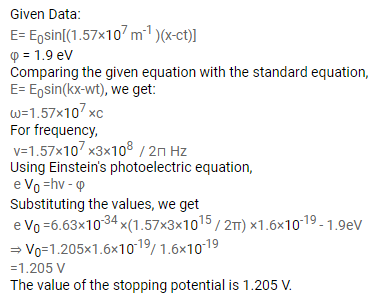# The electric field associated with a light wave is given

Question:

The electric field associated with a light wave is given byE $=E_{0} \sin \left(1.57 \times 10^{7} \mathrm{~m}^{-1}\right)(x-c t)$. Find the stopping potential when this light is used in an experiment on photoelectric effect with the emitter having work function $1.9 \mathrm{eV}$.

Solution: